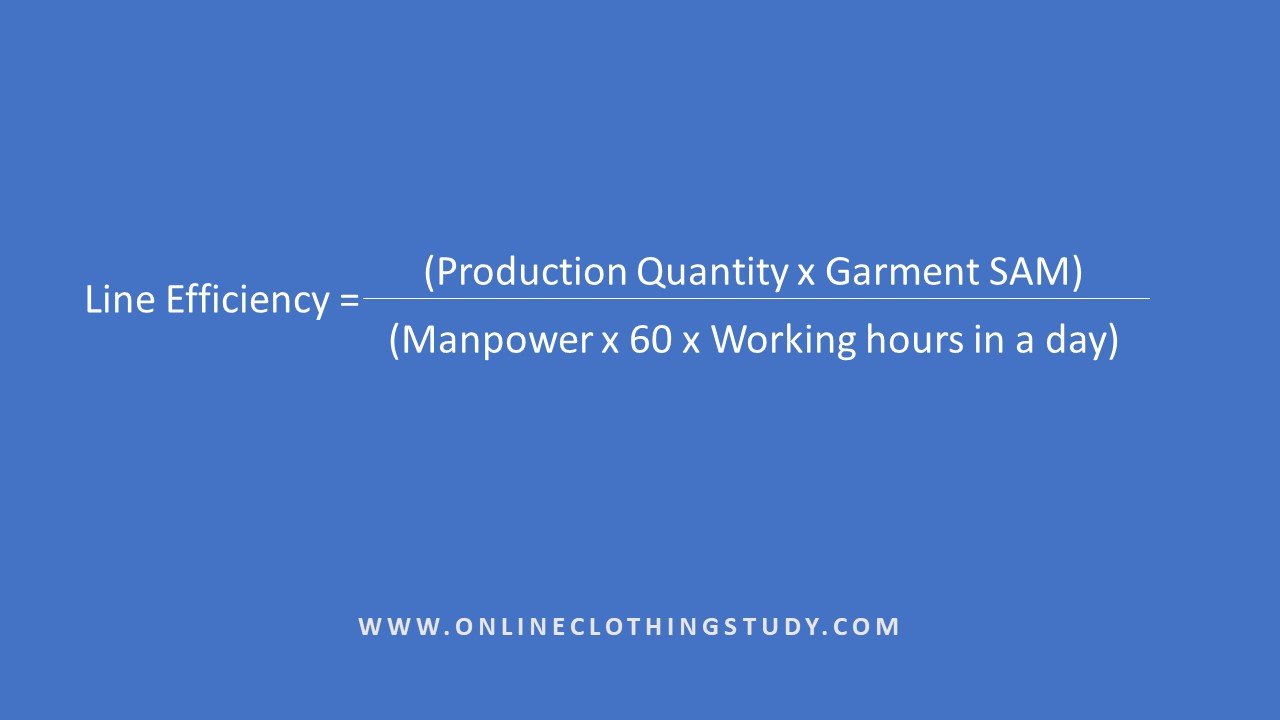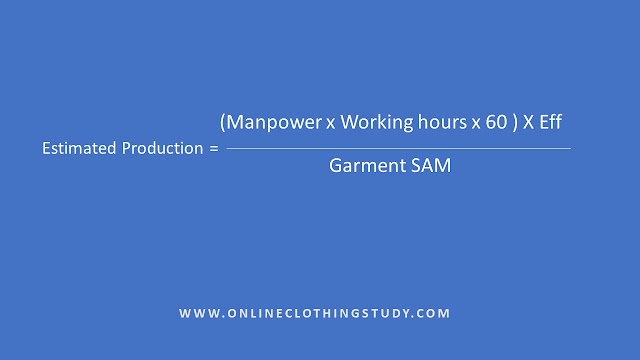# Line efficiency and produced quantity – which one comes first?

Question:
For calculating line efficiency, we require the number of pieces produced in a day, and for calculating the number of produced pieces in a day we required line efficiency. How it's possible? Confused!

This is a valid point. When someone reads the steps for calculating line efficiency and steps for calculating daily production capacity or estimated production for a future date in the same article (in a guide), he/she will be confused at the first time.

The same happened with the reader who asked for clarification on this. In our today’s post, I will clarify the calculation and data source for calculating these two performance KPIs.

Remember, both the following statements are correct in terms of formula.

1. To calculate line efficiency, we use daily production data (number of pieces produced) and
2. To calculate production capacity, we use line efficiency data.

Which formula to use - depends on what do you aim to calculate and measure depending on the situation.

A planner needs to know the estimated production quantity for the coming days. A line supervisor needs to know his/her line efficiency at the end of the day. These are two different requirements.

Either you need to calculate line efficiency or estimate to be produced garment quantity.

To know the actual production quantity data of a line, don’t depend on the formula or on any calculations. The actual production done by a line is real. Get that data from the line production recorder or from the line supervisor. If you have a production tracking system, you will get actual production data from the system (computer).

### Here is an example of line efficiency calculation.

Let's say, you need to calculate today's line efficiency and you know today's production quantity of your factory by line. Actual production data is available once your line produced garments. Now you need to calculate the efficiency of a line. To calculate the line efficiency, you need to use daily production data. And the formula used for calculating line efficiency is

Line efficiency= ((Production quantity x Garment SAM)/ (Manpower x 60 x Shift hours))x100

In the above formula, you need to use the actual production quality, not the estimated one.

Using the same method, you can calculate previous days' line efficiency from the daily production data. If you are keeping a daily production log, you might have date-wise production data.

You can use the OCS line efficiency calculator for calculating line efficiency.

### Calculating number of pieces produced (estimated value)

In the second case, you need to know the expected daily production in the coming days. Let's say you want to estimate tomorrow's production quantity for a line. Garments are not made yet.

How will you get that? In this situation, you need to use average line efficiency data (historical data) to calculate the estimated production quantity. With the average line efficiency data, you need some other information – expected manpower, style SAM.

The formula is shown in the slides below.To be more accurate in the expected daily production quantity you need to consider the learning curve efficiency. Consider employee absenteeism and product types.

Related post: Relation between order quantity and line efficiency in garment production